# Algebra + area - math problems

#### Number of problems found: 539

• ABS trianglePoint S is the center of the square ABCD. The ABS triangle has a content of 9 cm2. What is the circumference of the square in cm?
• Squares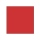From the square with the integer side, cut out the square with the integer side so that the residual area is 100. What is the longest possible side of the larger square?
• Trapezoid and diagonals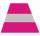Find the area of a trapezoid with bases a = 24 cm, c = 16 cm and diagonals u = 22 cm, v = 26 cm.
• The length 8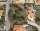The length of a rectangular field is x + 4 and its width is x + 2. what is the area of the field? Answer in polynomials expressions.
• What is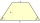What is the circumference of an isosceles trapezoid with a content of 106.75 cm 2 , the lengths of the sides are in the ratio 1: 3: 2: 1 and the bases are 6.1 cm apart?
• Height of the prism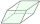The volume of the quadrilateral prism is 723.6 cm3. The base of this prism is a rhombus with a side 9 cm long and a corresponding height of 6.7 cm long. Find the height of the prism.
• 144 stepsTo get around the garden we have to take 144 steps. 8 steps less in width than in length. One step is 5 decimetres long. What are the dimensions of the garden? What is its perimeter and its area?
• The widthThe width of a rectangular garden is 4 m less than the length. If the area of a rectangular garden is 96 square meters, what is the dimension of the garden?
• Semicircle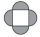The ornament consists of one square and four dark semicircles. The area of the square is 4 cm ^ 2. Find the area of one dark semicircle and round the result to hundreds.
• The isoscelesThe isosceles trapezoid ABCD has bases of 18 cm and 12 cm. The angle at apex A is 60°. What is the circumference and content of the trapezoid?
• A trapezoid 3A trapezoid ABCD has the bases length of a = 120 mm, c = 86 mm and the area A = 2,575 mm2. Find the height of the trapezoid.
• TrapezoidsIn the isosceles trapezoid ABCD we know: AB||CD, |CD| = c = 8 cm, height h = 7 cm, |∠CAB| = 35°. Find the area of the trapezoid.
• Perimeter to area 2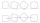Find the area of a square whose perimeter is 100 cm with solve.
• The area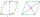The area of a rhombus is 143 m2. If the longer diagonal is 26 m, find the shorter diagonal in inches named d1.
• A rectangular 3A rectangular plot of land has an area of 3/2 square kilometers and a length of ¾ kilometer.  What is the width of the plot of land?
• Louis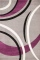Louis wants to carpet the rectangular floor of his basement. The basement has an area of 5,120 square feet. The width of the basement is 4/5 its length. What is the length of Louis's basement?
• Base side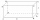In a quadrilateral prism, are known surface area S = 12400 mm2 base side m = 40mm and prism height = 120mm, what is the length of base side n =?
• Parallelogram diagonalsFind the area of a parallelogram if the diagonals u1 = 15 cm, u2 = 12 cm and the angle formed by them is 30 degrees.
• Center of gravity and medianIn the isosceles triangle ABC, the center of gravity T is 2 cm from the base AB. The median parallel to the AB side measures 4 cm. What is the area of the ABC triangle?
• Poisson distribution - daisies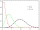The meadow behind FLD was divided into 100 equally large parts. Subsequently, it was found that there were no daisies in ten of these parts. Estimate the total number of daisies in the meadow. Assume that daisies are randomly distributed in the meadow.

Do you have an exciting math question or word problem that you can't solve? Ask a question or post a math problem, and we can try to solve it.

We will send a solution to your e-mail address. Solved examples are also published here. Please enter the e-mail correctly and check whether you don't have a full mailbox.

Algebra - math problems. Area - math problems.